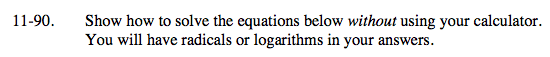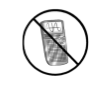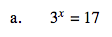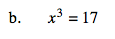### Home > A2C > Chapter 11 > Lesson 11.2.4 > Problem11-90

11-90.Take the log of both sides.

$\log (3^\textit{x})=\log (17)$

Apply the Power Property of Logarithms.

x · log(3) = log(17)

$\textit{x}= \frac{ \log(17) }{ \log(3)}$Find the cubic root of both sides of the equation.

$\textit{x}= \sqrt{ 17 }$